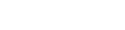• Home
• Matrices and Determi...

# Matrices and Determinants Questions for DU JAT and IPMAT

Author : Palak Khanna

March 3, 2022

SHARE

Matrices and determinants topic is a part of mathematics that deals with linear equations. A matrix is a group of numbers used to express linear equations, while a determinant is a unique number related to that matrix.

Also, you can perform mathematical operations on matrices such as addition, subtraction, and multiplication. You can expect 4-5 questions based on this topic in competitive exams like DU JAT and IPMAT.

This post shall guide you through the important Matrices and Dertimants Questions for DU JAT and IPMAT.

## Matrices and Determinants Question and Answers for DU JAT and IPMAT

Most of you might face difficulties while solving these questions. However, learning a few simple techniques would help you solve these questions in a matter of seconds.

We have provided a few samples here to help you understand the Questions asked in IPMAT and DU JAT from matrices and determinants topics.

Question 1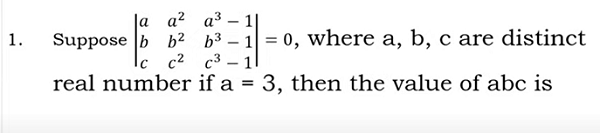Before start answering this question, represent the determinant in the following way.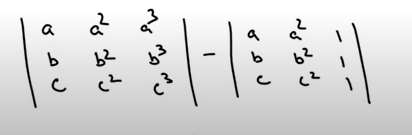Remove a,b,c from both the matrices.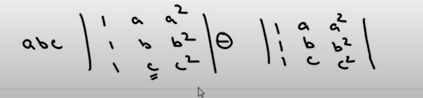Then remove common values such as a,b,c and 1 from the above matrices. Then, it becomes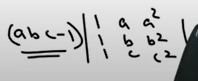Equate (abc-1) value = 1

abc = 1

Question 2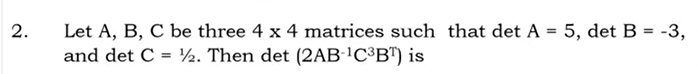To solve this question, you must know the following formulas: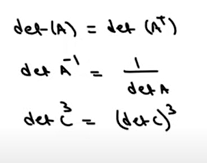Remove 2 from the expression, then it becomes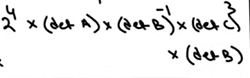As per the given question, Determinant of A = 5

Determinant of B = 3

Determinant of C = 1/2

=16X5X1/-3X1/8X-3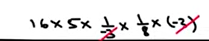=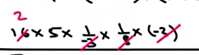= 10

Question 3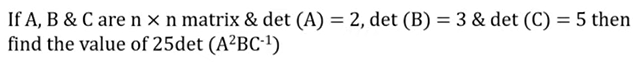In this question, you need to find the value of the following expression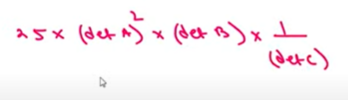Determinant of A power 2 = 4

Determinant of B = 3

Determinant of C inverse = 1/5

Therefore, the expression becomes as follows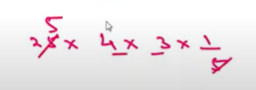= 5 X 4 X 3X 1

The final value = 60

Question 4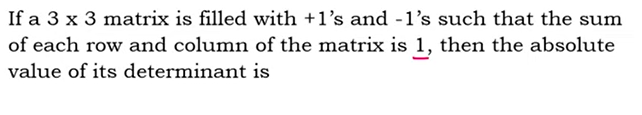Let us consider the 3X3 matrix as given belowThe value of its determinant becomes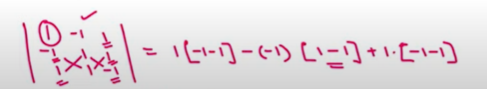= - 2 + 0 - 2

= -4

Therefore the determinant of - 4 = 4

Question 5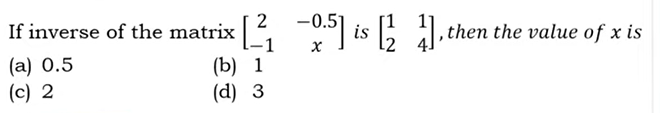You must know that any matrix multiplied with its inverse matrix becomes an Identity Matrix.

For Example: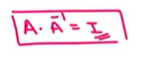Apply the same formula for the given expression. Then it becomes as follows:-1+2x=0

2x=1

x=1/2

x=0.5

• Home
• Matrices and Determi...

# Matrices and Determinants Questions for DU JAT and IPMAT

Author : Palak Khanna

Updated On : March 3, 2022

SHARE

Matrices and determinants topic is a part of mathematics that deals with linear equations. A matrix is a group of numbers used to express linear equations, while a determinant is a unique number related to that matrix.

Also, you can perform mathematical operations on matrices such as addition, subtraction, and multiplication. You can expect 4-5 questions based on this topic in competitive exams like DU JAT and IPMAT.

This post shall guide you through the important Matrices and Dertimants Questions for DU JAT and IPMAT.

## Matrices and Determinants Question and Answers for DU JAT and IPMAT

Most of you might face difficulties while solving these questions. However, learning a few simple techniques would help you solve these questions in a matter of seconds.

We have provided a few samples here to help you understand the Questions asked in IPMAT and DU JAT from matrices and determinants topics.

Question 1Before start answering this question, represent the determinant in the following way.Remove a,b,c from both the matrices.Then remove common values such as a,b,c and 1 from the above matrices. Then, it becomesEquate (abc-1) value = 1

abc = 1

Question 2To solve this question, you must know the following formulas:Remove 2 from the expression, then it becomesAs per the given question, Determinant of A = 5

Determinant of B = 3

Determinant of C = 1/2

=16X5X1/-3X1/8X-3== 10

Question 3In this question, you need to find the value of the following expressionDeterminant of A power 2 = 4

Determinant of B = 3

Determinant of C inverse = 1/5

Therefore, the expression becomes as follows= 5 X 4 X 3X 1

The final value = 60

Question 4Let us consider the 3X3 matrix as given belowThe value of its determinant becomes= - 2 + 0 - 2

= -4

Therefore the determinant of - 4 = 4

Question 5You must know that any matrix multiplied with its inverse matrix becomes an Identity Matrix.

For Example:Apply the same formula for the given expression. Then it becomes as follows:-1+2x=0

2x=1

x=1/2

x=0.5